# How to use BODMAS (BIDMAS / PEMDAS)

How to use BODMAS (BIDMAS / PEMDAS)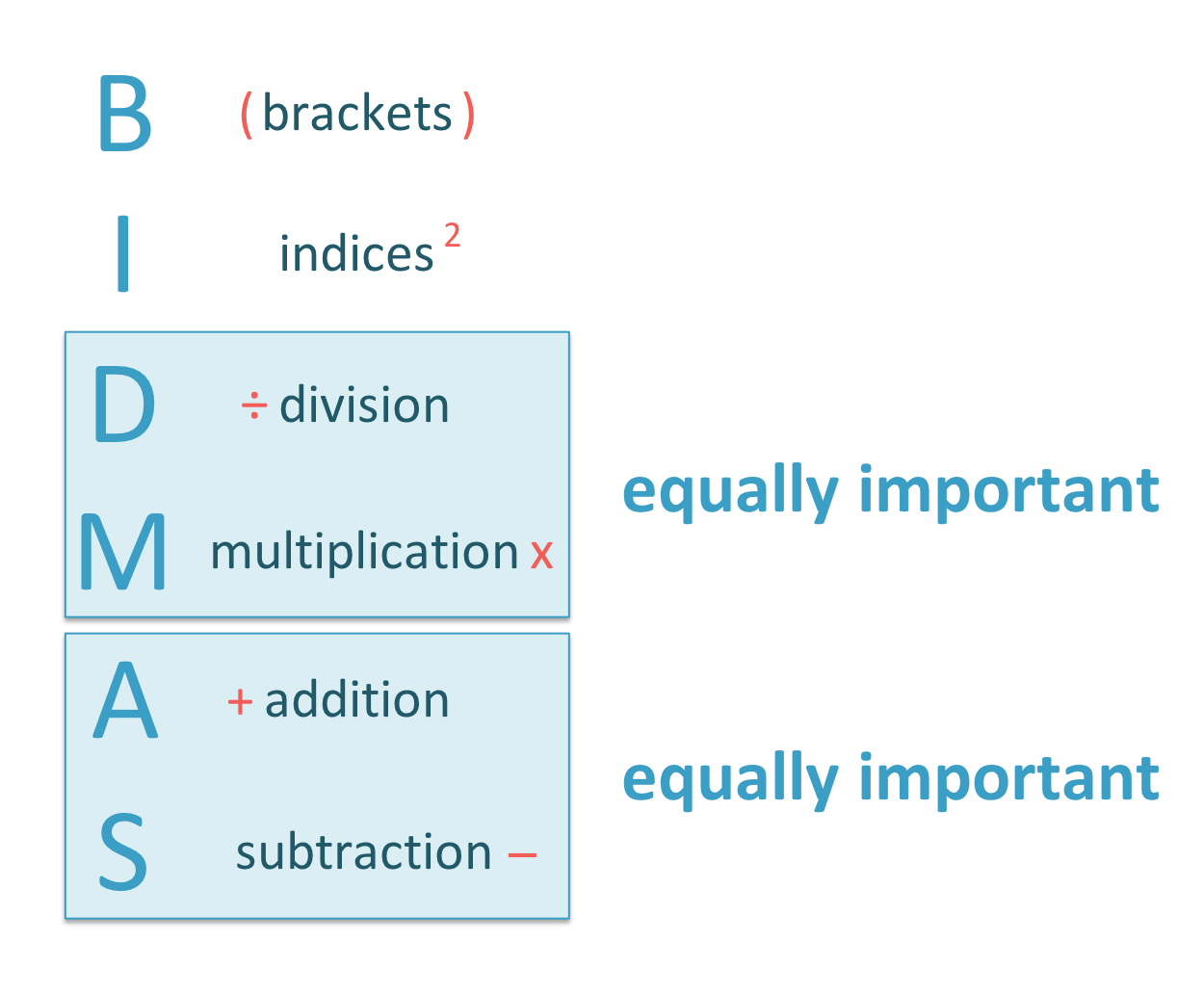• BIDMAS tells us the order in which to do the operations in a calculation, starting with brackets and ending with subtraction.
• ‘B’ stands for Brackets.
• ‘I’ stands for Indices.
• ‘D’ stands for Division.
• ‘M’ stands for Multiplication.
• ‘S’ stands for Subtraction.
• Division and multiplication are equally important in order.
• Addition and subtraction are also equally important in order.
BIDMAS / BODMAS are used to decide what order a calculation should be done.• There are no brackets in this sum and no indices, so we move on to division and multiplication.
• Division and multiplication have the same importance, so we do both of these operations now.
• 4 × 9 = 36 and 25 ÷ 5 = 5.
• The sum 4 × 9 + 25 ÷ 5 becomes 36 + 5.
• 36 + 5 = 41 and so, 4 × 9 + 25 ÷ 5 = 41.Supporting Lessons

# BODMAS, BIDMAS and PEMDAS Explained

## What is BODMAS?

BODMAS stands for brackets, order, division, multiplication, addition and subtraction. BODMAS tells us the order in which to do a calculation. Start with the brackets and end with addition and subtraction.BODMAS stands for the following order of operations:

• B stands for brackets.
• O stands for order.
• D stands for division.
• S stands for subtraction.
• For example, here is 4 + 2 × 5.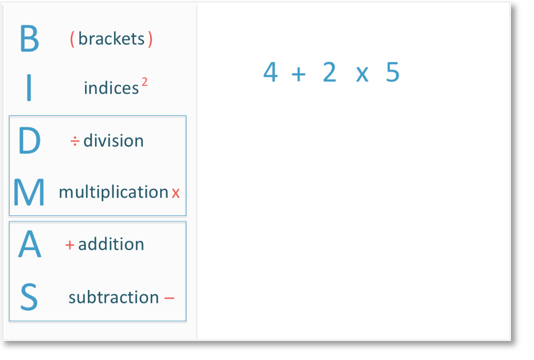We follow the order of BODMAS. There are no brackets in this example so we move on. There are no indices or divisions either. We have a multiplication so we do the multiplication first.2 × 5 = 10. Now we can do the additon.4 + 10 = 14 and so, 4 + 2 × 5 = 14.

The order of operations matters because it is important that a mathematical calculation is understood in the same way by everybody. Without an order, different answers could be found for the same calculation.

If we simply read from left to right, 4 + 2 × 5 would give us a different answer, which would be wrong. We would do 4 + 2 = 6 and then multiply this by 5 to get 30.

It is important that the rules of BODMAS are always followed in order to obtain the correct answer. The order of operations given by BODMAS are always used in mathematics.

The order of operations are shown in the following table:

Order Operation Meaning
1st B Brackets
2nd O Order
3rd D Division
M Multiplication
S Subtraction

## How to Use BODMAS

To use BODMAS, start by working out the calculations in the brackets, then calculate any indices, then look for any multiplications and divisions and work these out from left to right. Finally, do any additions and subtractions from left to right.

For example, here is 3 × (9 – 4). This example contains brackets and so, we do the calculation inside the brackets first.9 – 4 = 5 and so the calculation can be changed to say 3 × 5.

The multiplication is the only operation left so we work this out. 3 × 5 = 15. The answer to 3 × (9 – 4) = 15.

It is important to understand that multiplication and division have the same order and are done at the same time, from left to right.

Here is an example of using the BIDMAS rule to work out 4 × 9 + 25 ÷ 5.

There is no brackets or indices. The first operations on the BIDMAS list are division and multiplication.Multiplication and division have the same importance in the order of operations.

If there is a multiplication and division, simply work them both out, working from left to right.

From left to right, the multiplication comes before the division, so in this example, we do the multiplication first.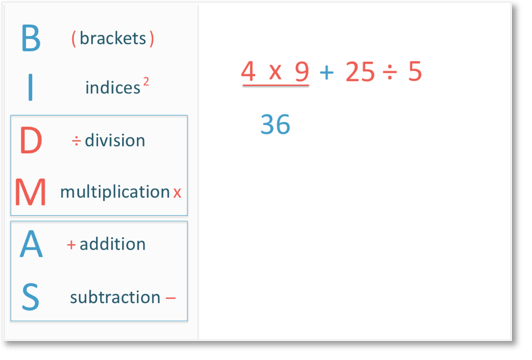4 × 9 = 36.

Then we do the division.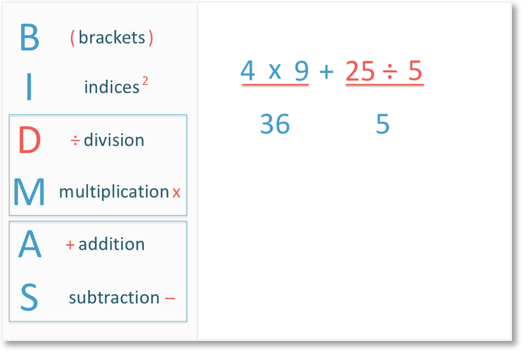25 ÷ 5 = 5.

In BIDMAS (or BODMAS), D and M come before A and S. The order of operations tells us to always do multiplication and division before addition and subtraction.

Finally, we add 36 and 5 to get 41.The answer to this calculation is 41.

When teaching the order of operations using BODMAS, it is helpful to write a new line of working out directly below each time a new operation has been calculated.Here is another example of using the BIDMAS (BODMAS) rule to work out 6 × 7 – 4 × 8.

The most simple rule of BODMAS is to make sure that addition and subtraction are the last operations to be calculated.

We perform multiplication before subtraction.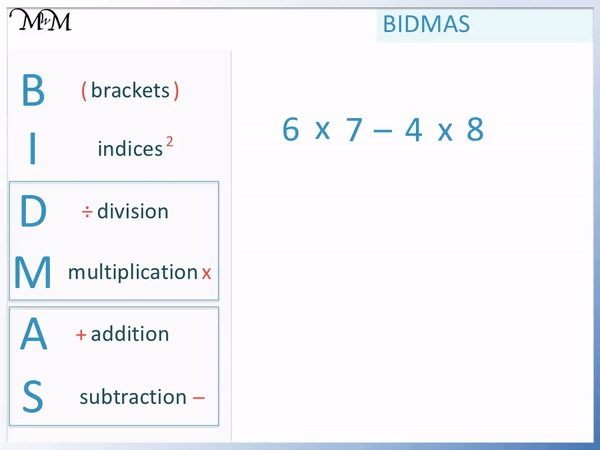6 × 7 = 42 and 4 × 8 = 32.

Finally we perform the subtraction. 42 – 32 = 10.

The answer to this BODMAS example is 10.

Here is an example of BIDMAS involving indices. If using BODMAS, then this is an example involving orders. We have 1 + 62 ÷ 4.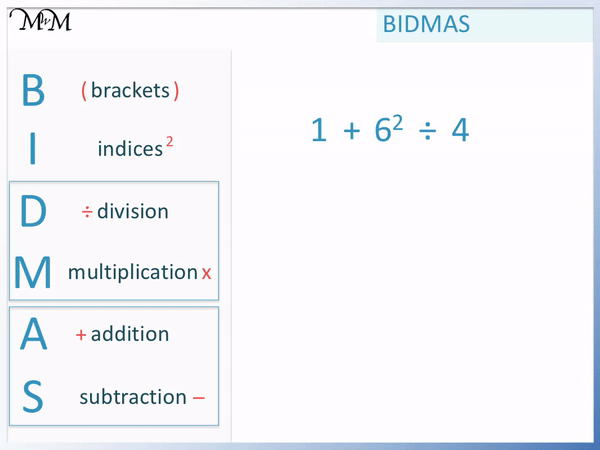There are no brackets and in the BIDMAS list, the next operation is indices. The 2 on the 62 is an indice. It means to square the 6, which means to multiply 6 by itself.

62 = 36.

We now have 1 + 36 ÷ 4.

According to BIDMAS, division is carried out before addition. 36 ÷ 4 = 9 and so, the calculation simplifies to 1 + 9, which equals 10.

1 + 62 ÷ 4 = 10.

The rules of BODMAS are:

• Work out the calculation inside any brackets first.
• Multiply and Divide before Adding and Subtracting.
• If there is only multiplication and division, work them out from left to right.
• If there is only addition and subtraction, work them out from left to right.

A common mistake when teaching BODMAS is that addition is more important in order than subtraction, whereas it actually has the same importance.

For example, in 10 – 2 + 3, we work from left to right. 10 – 2 = 8 and then 8 + 3 = 11. This is the correct answer.

However if we do addition before subtraction, we would do the 2 + 3 first to get 5. Then 10 – 5 = 5 and this give us the wrong answer.

The reason for this BODMAS mistake is that in the name BODMAS, it appears that A comes before S. This leads people to do additon before subtraction when in actual fact they have the same importance and should be worked out from left to right.

Here is the BIDMAS acronym shown with ‘multiplication and division’ and ‘addition and subtraction’ having the same importance. It is important to show these types of examples when teaching the order of operations.BODMAS is wrong if it is taught as meaning to divide before multiplying or to add before subtracting. Since multiplication and division have the same importance and addition and subtraction have the same importance, BODMAS could be written as BO(D/M)(A/S).

Here are some examples of using BODMAS with answers.

3 + 2 × 5 13 Multiplication before addition
(5 – 1) × 2 8 Work out brackets before multiplication
3 + 10 ÷ 2 8 Division before additon
20 – 10 + 6 16 Work left to right
20 – (10 + 6) 4 Work out brackets before subtraction
52 + 22 29 Work out orders before addition
(5 + 2)2 49 Work out brackets before orders

## What is the Difference Between BODMAS and BIDMAS?

BODMAS and BIDMAS are two different names to help remember the order of operations. The O in BODMAS stands for order and the I in BIDMAS stands for indices. Order and indices are just two different names for the same thing.The order of operations according to BIDMAS are:

Order Operation Meaning
1st B Brackets
2nd I Indices
3rd D Division
M Multiplication
S Subtraction

The order of operations according to PEMDAS are:

Order Operation Meaning
1st P Parentheses
2nd E Exponent
3rd M Multiplication
D Division
S Subtraction

There is also BEDMAS, where the E stands for exponent. Exponent is another word for indice or order.

It is important to understand that BIDMAS, BODMAS, BEDMAS and PEMDAS are the same thing. They are just different names for understanding the order of operations.

In maths, operations are the calculations such as addition, subtraction, multiplication and division. BODMAS, BIDMAS, BEDMAS and PEMDAS tell us the order in which to do the operations in a sum.

Using different combinations of the words brackets/parantheses, indices/order/exponent and switching the orders of D/M and A/S, we could also have the names BIDMSA, BIMDAS, BOMDAS, BODMSA, PEDMAS PODMAS etc.

There is no difference between BIDMAS, BODMAS, PEMDAS and all of the other names here apart from the name. They all refer to the order in which we should do our calculation.

## What are Indices in BIDMAS?

Indices are the small numbers written in the top right corner of another number and they mean to raise this number to a power. For example, 52 means 5 × 5 and the indice is the 2.

Other words used for indices include exponents and orders. Orders, exponents and indices are all different names for the power to which a number is raised.

Indices tell us how many times to multiply a number by itself.

Common indices seen in maths are squaring and cubing a number. Squaring a number means to multiply it by itself, such as 32, which means 3 × 3.

42 means 4 × 4 = 16.When teaching indices, it is a common mistake for someone to multiply the number by the indice. For example in 42, it is common to see people work out 4 × 2 instead of 4 × 4. As a result they get the wrong answer of 8 instead of the correct answer of 16.

When using BIDMAS or BODMAS, indices come up frequently and this is therefore a common mistake.

Here are some examples of using indices in BIDMAS (or orders in BODMAS).

We have 52 – 32.BIDMAS tells us to work out any indices before subtraction.

52 = 5 × 5 = 25.

32 = 3 × 3 = 9.

Now that we have worked out the indices, we subtract. 25 – 9 = 16.

Notice how this next example is a little different. It uses the same numbers but has brackets.

We have (5 – 3)2.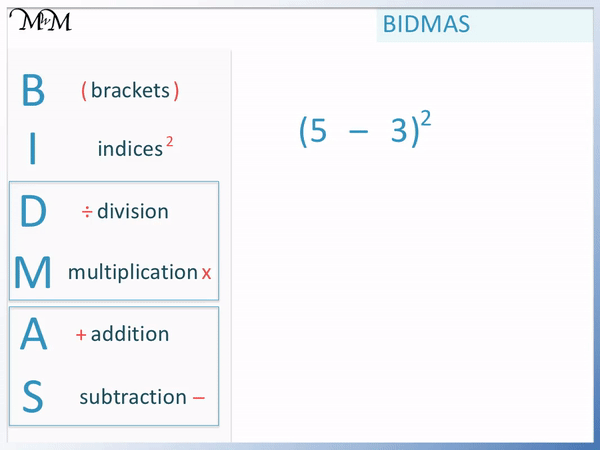Because we have brackets, we must work out the answer inside the brackets first. 5 – 3 = 2.

We replace (5 – 3) with 2 in the calculation to get 22. 2 × 2 = 4 and so, the answer is 4.

BODMAS can be used to make different answers using the same numbers. Simply use different combinations of brackets and indices to change the answer.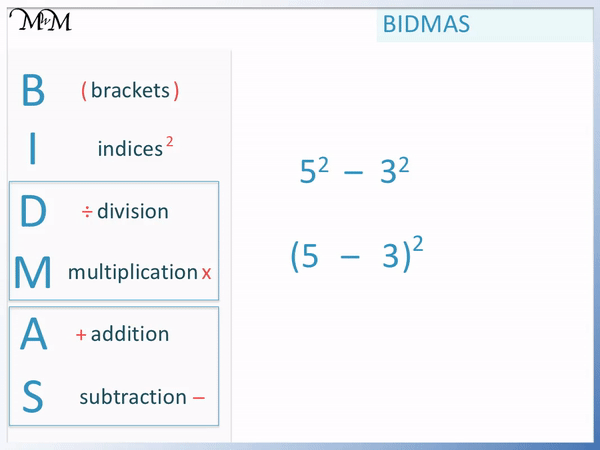The answer to the first sum is 16, whilst the answer to the second sum is 4. It is important to look for any brackets in a calculation and work these out first.Now try our lesson on How to Add and Subtract Negatives using a Number Line where we learn about negative numbers and introduce adding and subtracting negative numbers using a number line.